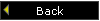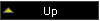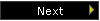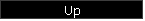# sFLC3 API Calls - A Concise ListNEW:

# sFLC3 API Calls - A Concise List

Debug programs should link to the debug version of the DLL (FLC3d.DLL) whereas release programs link to FLC3.DLL

A preliminary reading of our walk-through document is recommended.

## CFLC Class Level

### Notes

Express Pro
CFLC::CFLC(void)     Constructor X
int CFLC::Reset(void)   error code Clears all FLC components X
int CFLC::GetFLCNum(void)     Returns FLC serial number X
void CFLC::ResetFLCno(void)     Resets FLC counter (use with caution)   X
void CFLC::SetNumFuzzySets(int n) number of fuzzy sets   Sets number of Fuzzy Sets in FLC X
int CFLC::GetNumFuzzySets(void)   number of fuzzy sets   X
void CFLC::SetNumVars(int n) number of variables   Always 3 (in all existing DLL clients) X
int CFLC::GetNumVars(void)   number of variables   X
void CFLC::SetRuleSetSize(int n) number of rules     X
int CFLC::GetRuleSetSize(void)   number of rules   X
void CFLC::SetName(string strFLC) FLC name   Generated automatically on creation (FLC_nn where nn is the FLC number) X
string CFLC::Name(void)   FLC name   X
void CFLC::SetShape(int sn) FLC shape number   Only 2 shapes coded at present:
0 for 3x3, and 1 for 5x5
X
int CFLC::GetShape(void)   FLC shape number   X
void CFLC::SetRSModel(string strRSM) Rule Set model name     X
string CFLC::RSModel(void)   Rule Set model name   X
void CFLC::SetRSModel(int nRSn) rule set model number   Check FLC_DLL.H for acceptable values X
int CFLC::RSModelMax(void)   Max Rule Set Model Upper bound in predefined rule sets X
int CFLC::FSModelMax(void)   Max Fuzzy Set Model Upper bound in predefined fuzzy sets X
int CFLC::Calculate(double *Val)
int CFLC::Calculate(void);
FLC inputs (doubles) error code   X
int CFLC::DetermineRuleSetSize(void)   Number of Rules A fully determined rule set should be 9 for a 2-variable FL controller with each variable defined with 3 fuzzy states (or 25 if 5 fuzzy states). X
int CFLC::AllocateRuleSpace(void)   error code Used in Rule Set constitution X
int CFLC::InitRuleSet(int nSize)
int CFLC::InitRuleSet(void)
Number of rules error code SetRuleSetSize must have been called first if Size not given here. X
int CFLC::InitVarSet(int nNumVars) Number of variables error code   X
int CFLC::InitFuzzySets(int nNumFS) Number of fuzzy sets error code One can create many Fuzzy Sets, and associate/dissociate them from existing variables. For simplicity, our samples generally use 1 FS per Variable. X
int CFLC::ClearFuzzySets(void)
int CFLC::ClearVarSet(void)
int CFLC::ClearRuleSet()
error code Not often used separately. Use Reset() instead X
int CFLC::EvaluateAllPremises(void)
int CFLC::EvaluateAllPremises(double *Val)
Variable values error code See sample application for details

Only used with full access to API, otherwise called implicitly with Calculate call.
If values are not provided here, they must be defined prior to this call.

X
int CFLC::InferenceRules(void)   error code See sample application for details X
int CFLC::GenerateFuzzyOutputs(void)   error code See sample application for details X
double CFLC::OutState(int i) Fuzzy State error code Returns strength of fuzzy state "i" X
int CFLC::Defuzzify(int DeFuzz = 0)   error code Defuzzify outputs into a single "crisp" output.

Only one defuzzification method (fuzzy centroid) is coded in current version. It is probably the most balanced calculation method.

X
int CFLC::LoadDefault33RuleSet(CFLRule *rs, int NumRules, int ArrIdx)     Load one of the built-in 3x3 rule sets X
int CFLC::LoadDefault55RuleSet(CFLRule *rs, int NumRules, int ArrIdx)     Load one of the built-in 5x5 rule sets X
int CFLC::LoadCustom33RuleSet(CFLRule *rs, int ***CustRS33, string *CustRS33n, int NumRules, int ArrIdx)     Requires full access to the API   X
int CFLC::LoadCustom55RuleSet(CFLRule *rs, int*** CustRS55, string *CustRS55n, int NumRules, int ArrIdx)     Requires full access to the API   X
CFLVariable* VarArray()
CFuzzySet* FSArray()
CFLRule* RuleArray()
pointers These pointers allow the programmer to access the inner components of the FL controller. X
BOOL boolFS()
BOOL boolVS()
BOOL boolVS()
boolean Used to test whether the FLC has a FS (a Set of fuzzy sets), a VS or Variable Set, and a RS, i.e. a rule set. X
BOOL bFLC_Built()   boolean single call to check whether the FLC is operational X
int nCredit()   integer The DLL is enabled with a given credit, decremented when a new FLC is built. X
int nUsage()   integer Num FLC currently operational. X

To write your own FLC code, please refer to sample code, in particular the Sample1 console application which is particularly easy to read, and to the 22-page class description document.

Back to sFLC3Best viewed with MS Internet Explorer 5 and above